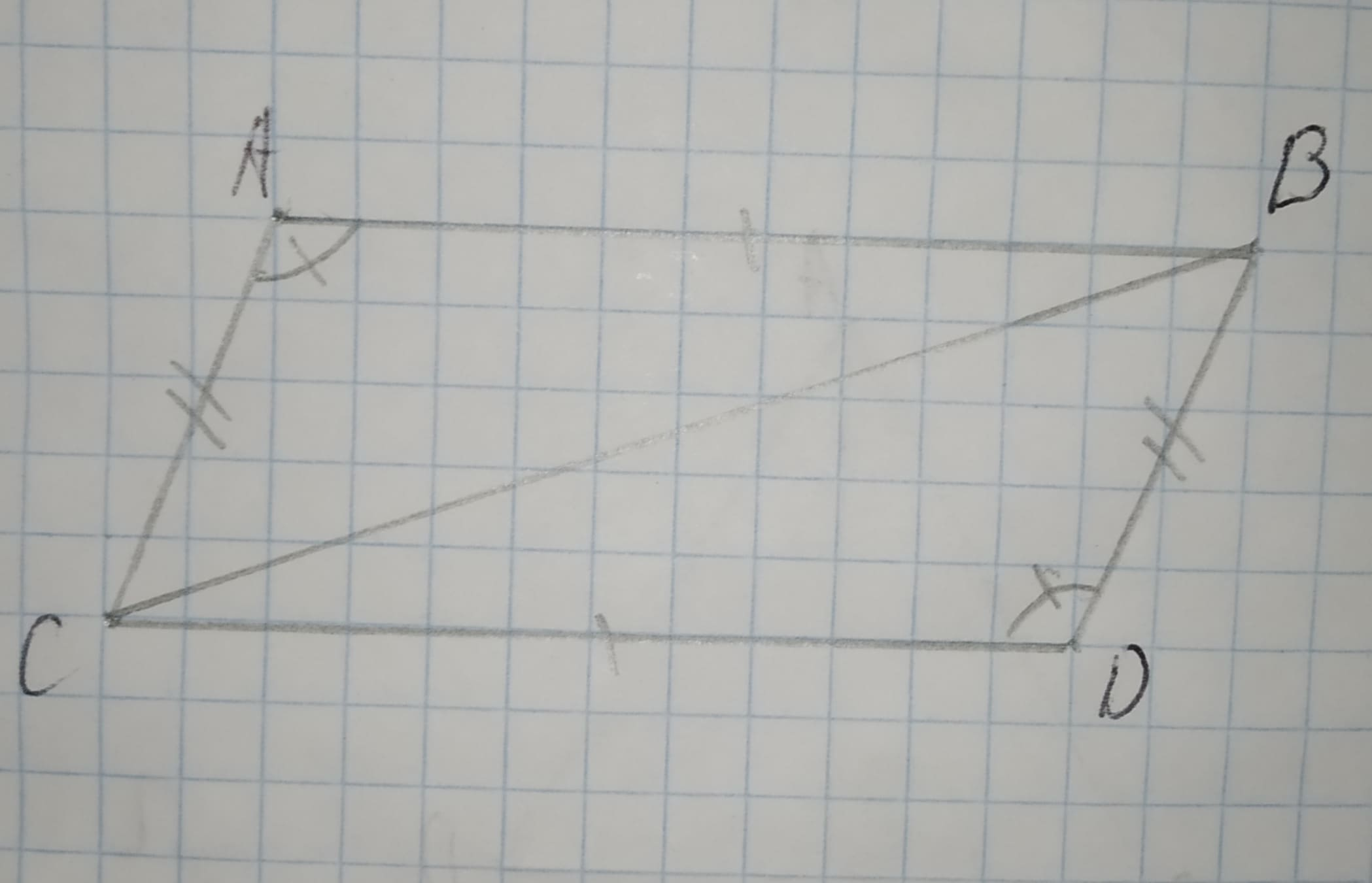# Select all statements that are true about the triangles. 12210203841.jpg -Triangle ABC and DCB are congruent by the Angle-Angle Triangle Congruence thdefazajx 2020-11-08 Answered

Select all statements that are true about the triangles.-Triangle ABC and DCB are congruent by the Angle-Angle Triangle Congruence theorem.
-Triangle ABC and BCD are congruent by the Angle-Side-Angle Triangle Congruence theorem.
-Triangle ABC and BCD are congruent by the Side-Side-Side Triangle Congruence theorem.
-Triangle ABC and DCB are congruent by the Side-Angle-Side Triangle Congruence theorem.
-Triangle ABC and DCB are congruent by the Side-Side-Side Triangle Congruence theorem.
-There is not enough information to determine if the triangles are congruent.

You can still ask an expert for help

## Want to know more about Congruence?

• Questions are typically answered in as fast as 30 minutes

Solve your problem for the price of one coffee

• Math expert for every subject
• Pay only if we can solve itAamina Herring
Step 1
We can see from the figure
AB=CD
AC=BD
BC=BC
So, by the SSS property of triangle.
We can say trainges ABC and DCB are congruent.
And also here $\mathrm{\angle }A=\mathrm{\angle }D$
So, by the side angle side property of triangle we can say ABC and DCB are congruent.
Step 2
Trainges ABC and DCB are congruent by the Side-Angle-Side triangle theorem.
Trainges ABC and DCB are congruent by the Side-Side-Side triangle theorem.